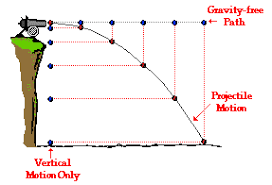## 4ESO FQ English 2018-2019. Unit 1 MOTION. Article 2.

Publicado: 07/10/2018 19:28 por easyfisquim en 4ESO FQ (10th gr Science) 2018-2019UNIT 1 :    MOTION

SECTION 2 , LESSON 1,  MRUA.
( Moviment rectilini uniformement accelerat, Constant or uniform acceleration motion ).

-  Converting units with conversion factors review.

( Do the unit changes suggested in class ).
( Corrections of the exercises suggested ).

- Read the following description about whats the difference between velocity and acceleration, check also the corresponding equations and try to summarize the main issues at your notebook. Write it down if you agree with it too  :

The difference between velocity and acceleration can be drawn clearly on the following grounds:

The velocity of an object refers to the speed in a specific direction.
Velocity is nothing but the rate of change of displacement. On the other hand, acceleration is the rate of change of velocity  with respect to time.

SCAFF 6 : - Traffic accident pic and talking.
File with scaffolding material to talk about the pic.

- Example 4 pag 23.

- Make and achieve exercises 8 and 9 on page 23.         Solutions :          ex8             ex9

Teachers paper with first MRUA equations proof.   X=Xo+Vo.t+1/2a.t^2

* Second important equation demonstration.    V^2-Vo^2=2.a.(X-Xo)

- Example 5 page 24.

* Useful links to check to review constant acceleration motion lesson :

- MRUA link 1  ( Video explaining MRUA and main MRUA equations and graphs ).

- MRUA link 2  ( Several links and videos working on MRUA main issues ).

- MRUA link 3  ( Acceleration and gravity, linked equations, exercises and examples ).

- Exercises 43, 44 and 56 and 56end pag 34 and 35.

- Homework to do for next monday :  ex 45, 46 and 47 on page 34.

- Exercise MRUA to achieve , quadratic equation to get time.

SCAFF 7 :- MRUA graphs text to fill out.  1 , 2 and 3.  ------   Solutions :  1  ,  2   and   3.

-  Lets do this one now:       Exercise to do about working out the time to go over certain
distance in MRUA.

A car starts its movement with a u.r.a.m (MRUA) motion. Its showing an acceleration of
12m/s2.  How much time will it take to cover or go over 625m?.

Solution:  Check the solution HERE

+ In this Link you have a great help with a website provided in order to be able to create any kind of graph
you need to show your data ( bar graphs, line graphs, pie charts...).  Just choose your desing, color,
back ground and introduce your data and the labels you want to appear on the chart :

- Homework  :

Look over the   Example 6 pag 26.  where several stages from a MRUA motion are described.
Your task for next monday is to prepare an outline or a text using similar explanations but in english to
describe whats happening at the graph but making use of your own words and expressions.
Then copy the graph, check the scaffolding  material provided on the last lesson to write your sentences
and organize the paragraphs to explain what is shown at the graph and what the numerical calculations
and results mean. Prepare a single separated sheet from your workbook to hand it in to your teacher.

SCAFF 7b : Check this FILE to get some help to do the homework activity above.

SCAFF 7c :  Check this FILE to fill out 2 grids which will help you a lot to write your essay or writing

- Do now exercises 51, 52 and 53 on page 34 and later exercise 57 on page 35.

Solutions :           ex 51 part1  ;   ex 51 part 2

ex 52 and 53 on page 34

ex 57 on page 35

Comentarios  Ir a formulario